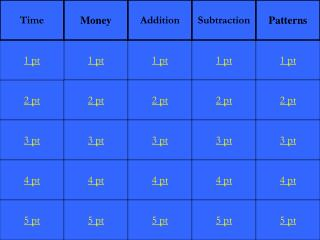DownloadDownload Presentation2 pt

# 2 pt

Download Presentation## 2 pt

- - - - - - - - - - - - - - - - - - - - - - - - - - - E N D - - - - - - - - - - - - - - - - - - - - - - - - - - -
##### Presentation Transcript

1. Time Money Addition Subtraction Patterns 1 pt 1 pt 1 pt 1 pt 1 pt 2 pt 2 pt 2 pt 2 pt 2 pt 3 pt 3 pt 3 pt 3 pt 3 pt 4 pt 4 pt 4 pt 4 pt 4 pt 5 pt 5 pt 5 pt 5 pt 5 pt

2. The time on the clock

3. What is 3:00

4. The time on the clock

5. What is 9:00

6. The time on the clock

7. What is 4:30

8. The time on the clock

9. What is 11:30

10. The time on the clock

11. What is 7:15

12. The total value of the money

13. What is 25 ¢

14. The total value of the money

15. What is 75 ¢

16. The total value of the money

17. What is 45 ¢

18. The total value of the money

19. What is 41 ¢

20. The total value of the money

21. What is 85 ¢

22. 4 + 3 =

23. 9 + 5 =

24. What is 14

25. 12 + 3 =

26. What is 15

27. 13 + 7 =

28. What is 20

29. 18 + 9 =

30. What is 27

31. 9 - 5 =

32. 8 - 3 =

33. 18 - 4 =

34. What is 14

35. 14 - 6 =

36. 49 - 13 =

37. What is 36

38. Write the next 2 numbers in the pattern 2, 4, 6, 8, __, __

39. What are 10, 12

40. Write the next 2 numbers in the pattern 3, 6, 9, 12, __, __

41. What are 15, 18

42. Write the next 2 numbers in the pattern 5, 15, 25, __, __

43. What are 35, 45

44. Write the next 2 numbers in the pattern 1, 3, 5, 7, __,__

45. What are 9, 11

46. Write the next 2 numbers in the pattern 4, 8, 12, __, __